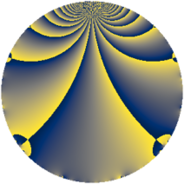# Properties

 Label 475.2.aLevel $475$ Weight $2$ Character orbit 475.a Rep. character $\chi_{475}(1,\cdot)$ Character field $\Q$ Dimension $28$ Newform subspaces $10$ Sturm bound $100$ Trace bound $2$

# Related objects

## Defining parameters

 Level: $$N$$ $$=$$ $$475 = 5^{2} \cdot 19$$ Weight: $$k$$ $$=$$ $$2$$ Character orbit: $$[\chi]$$ $$=$$ 475.a (trivial) Character field: $$\Q$$ Newform subspaces: $$10$$ Sturm bound: $$100$$ Trace bound: $$2$$ Distinguishing $$T_p$$: $$2$$

## Dimensions

The following table gives the dimensions of various subspaces of $$M_{2}(\Gamma_0(475))$$.

Total New Old
Modular forms 56 28 28
Cusp forms 45 28 17
Eisenstein series 11 0 11

The following table gives the dimensions of the cuspidal new subspaces with specified eigenvalues for the Atkin-Lehner operators and the Fricke involution.

$$5$$$$19$$FrickeDim.
$$+$$$$+$$$$+$$$$6$$
$$+$$$$-$$$$-$$$$8$$
$$-$$$$+$$$$-$$$$9$$
$$-$$$$-$$$$+$$$$5$$
Plus space$$+$$$$11$$
Minus space$$-$$$$17$$

## Trace form

 $$28 q + q^{2} - 2 q^{3} + 29 q^{4} - 3 q^{7} + 9 q^{8} + 16 q^{9} + O(q^{10})$$ $$28 q + q^{2} - 2 q^{3} + 29 q^{4} - 3 q^{7} + 9 q^{8} + 16 q^{9} - 3 q^{11} - 6 q^{13} + 23 q^{16} - 3 q^{17} + 29 q^{18} - 2 q^{19} - 6 q^{21} + 12 q^{22} + 12 q^{23} + 8 q^{24} + 6 q^{26} - 8 q^{27} - 6 q^{28} - 16 q^{31} + 9 q^{32} - 6 q^{33} - 10 q^{34} - 27 q^{36} - 16 q^{37} + 3 q^{38} - 28 q^{39} + 4 q^{41} - 16 q^{42} + q^{43} - 2 q^{44} + 4 q^{46} + 15 q^{47} - 40 q^{48} - 13 q^{49} - 2 q^{51} - 30 q^{52} - 18 q^{53} - 52 q^{54} - 32 q^{56} + 2 q^{57} - 2 q^{58} + 2 q^{59} - 21 q^{61} - 48 q^{62} + 13 q^{63} + 79 q^{64} - 36 q^{66} + 20 q^{67} - 8 q^{68} + 18 q^{71} + 65 q^{72} - 23 q^{73} - 2 q^{74} - 5 q^{76} + 35 q^{77} - 24 q^{79} + 16 q^{81} + 2 q^{82} + 20 q^{83} - 56 q^{84} + 40 q^{86} + 76 q^{87} + 16 q^{88} + 2 q^{89} - 24 q^{91} + 20 q^{92} + 48 q^{93} + 24 q^{94} - 52 q^{96} - 58 q^{97} - 23 q^{98} + 9 q^{99} + O(q^{100})$$

## Decomposition of $$S_{2}^{\mathrm{new}}(\Gamma_0(475))$$ into newform subspaces

Label Dim $A$ Field CM Traces A-L signs $q$-expansion
$a_{2}$ $a_{3}$ $a_{5}$ $a_{7}$ 5 19
475.2.a.a $1$ $3.793$ $$\Q$$ None $$-1$$ $$0$$ $$0$$ $$2$$ $-$ $-$ $$q-q^{2}-q^{4}+2q^{7}+3q^{8}-3q^{9}-4q^{11}+\cdots$$
475.2.a.b $1$ $3.793$ $$\Q$$ None $$0$$ $$2$$ $$0$$ $$1$$ $+$ $-$ $$q+2q^{3}-2q^{4}+q^{7}+q^{9}+3q^{11}+\cdots$$
475.2.a.c $1$ $3.793$ $$\Q$$ None $$1$$ $$0$$ $$0$$ $$-2$$ $-$ $-$ $$q+q^{2}-q^{4}-2q^{7}-3q^{8}-3q^{9}-4q^{11}+\cdots$$
475.2.a.d $3$ $3.793$ $$\Q(\zeta_{14})^+$$ None $$-4$$ $$-2$$ $$0$$ $$0$$ $+$ $+$ $$q+(-1-\beta _{1})q^{2}+(-\beta _{1}+\beta _{2})q^{3}+(1+\cdots)q^{4}+\cdots$$
475.2.a.e $3$ $3.793$ 3.3.169.1 None $$-2$$ $$-2$$ $$0$$ $$-4$$ $-$ $-$ $$q+(-1+\beta _{1})q^{2}+(-1-\beta _{2})q^{3}+(2+\cdots)q^{4}+\cdots$$
475.2.a.f $3$ $3.793$ 3.3.148.1 None $$-1$$ $$-2$$ $$0$$ $$0$$ $+$ $+$ $$q-\beta _{1}q^{2}+(-1+\beta _{1}+\beta _{2})q^{3}+(\beta _{1}+\cdots)q^{4}+\cdots$$
475.2.a.g $3$ $3.793$ 3.3.169.1 None $$2$$ $$2$$ $$0$$ $$4$$ $+$ $-$ $$q+(1-\beta _{1})q^{2}+(1+\beta _{2})q^{3}+(2-\beta _{1}+\cdots)q^{4}+\cdots$$
475.2.a.h $3$ $3.793$ $$\Q(\zeta_{14})^+$$ None $$4$$ $$2$$ $$0$$ $$0$$ $-$ $+$ $$q+(1+\beta _{1})q^{2}+(\beta _{1}-\beta _{2})q^{3}+(1+2\beta _{1}+\cdots)q^{4}+\cdots$$
475.2.a.i $4$ $3.793$ 4.4.11344.1 None $$2$$ $$-2$$ $$0$$ $$-4$$ $+$ $-$ $$q-\beta _{2}q^{2}+\beta _{3}q^{3}+(1+\beta _{1}-\beta _{2})q^{4}+\cdots$$
475.2.a.j $6$ $3.793$ 6.6.66064384.1 None $$0$$ $$0$$ $$0$$ $$0$$ $-$ $+$ $$q-\beta _{4}q^{2}+(-\beta _{1}-\beta _{5})q^{3}+(1-\beta _{3}+\cdots)q^{4}+\cdots$$

## Decomposition of $$S_{2}^{\mathrm{old}}(\Gamma_0(475))$$ into lower level spaces

$$S_{2}^{\mathrm{old}}(\Gamma_0(475)) \cong$$ $$S_{2}^{\mathrm{new}}(\Gamma_0(19))$$$$^{\oplus 3}$$$$\oplus$$$$S_{2}^{\mathrm{new}}(\Gamma_0(95))$$$$^{\oplus 2}$$